GAN自提出以来就以生成对抗为目标进行模型优化，这种对抗真的区分了真实数据和生成数据了吗？ICLR2020中的一篇Real or Not Real, that is the Question（RealnessGAN）提出了对传统GAN判别数据真假的质疑，文章抛出了在传统GAN中对于判别器的鉴别输出为一常数score是不合理的观点，并由此提出合理的解决方案，本文将对RealnessGAN进行解析，探究GAN是否真的区分了真假。

# 论文引入

GAN自提出就得到了空前反响，GAN在逼真的图像生成方面以及处理高维数据时的灵活性上推动着包括图像，音频和视频生成的发展，更甚者在诸如强化学习、迁移学习等也展示了一定的价值。GAN通过生成器和判别器的博弈下达到交替更新，其核心就是模型能否鉴别数据的真实与生成并与生成器形成对抗。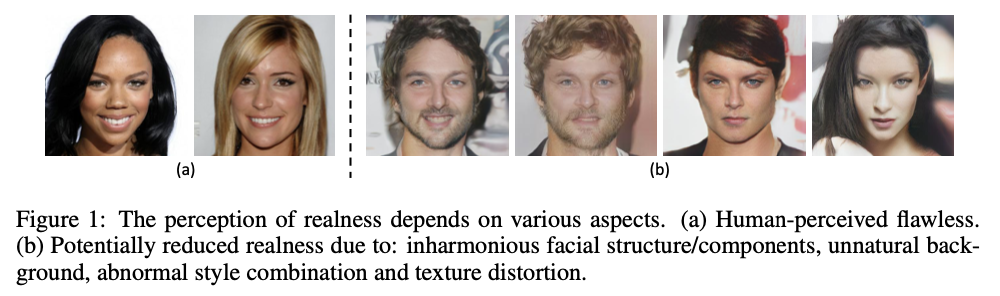• 将标量真实性得分扩展为分布的真实性得分，判别器$D$为生成器$G$提供了更强的指导。
• RealnessGAN的思路可以推广到一般的GAN中，具有较强的灵活性。
• 基于一个简单的DCGAN架构，RealnessGAN可以成功地从头开始学习生成$1024 \times 1024$分辨率的逼真的图像。

# RealnessGAN原理分析

\begin{equation} \begin{aligned} \min_G\max_DV(G,D)&=\mathbb E_{x \sim p_{data}}[\log D(x)]+E_{z \sim p_z}[\log (1−D(G(z)))] \\ &= \mathbb E_{x \sim p_{data}}[\log D(x)-0]+E_{x \sim p_g}[\log(1−D(x))] \end{aligned} \end{equation}

$\max_G\min_DV(G,D)= \mathbb E_{x \sim p_{data}}[\mathcal D_{KL}(\mathcal A_1 \Vert D(x))]+E_{x \sim p_g}[\mathcal D_{KL}(\mathcal A_0 \Vert D(x))]$

\begin{equation} \begin{aligned} \min_DV(G,D) &= \mathbb E_{x \sim p_{data}}[\mathcal D_{KL}(\mathcal A_1 \Vert D(x))]+E_{x \sim p_g}[\mathcal D_{KL}(\mathcal A_0 \Vert D(x))]\\ &= \int_x (p_{data}(x) \int_u \mathcal A_1(u) \log \frac{\mathcal A_1(u)}{D(x,u)}du + p_{g}(x) \int_u \mathcal A_0(u) \log \frac{\mathcal A_0(u)}{D(x,u)}du)dx \\ &= \int_x p_{data}(x) \int_u \mathcal A_1(u) \log \mathcal A_1(u)dudx + \int_x p_{g}(x) \int_u \mathcal A_0(u) \log \mathcal A_0(u)dudx\\ &- \int_x \int_u (p_{data}(x)\mathcal A_1(u)+p_g(x)\mathcal A_0(u)) \log D(x,u)dudx \end{aligned} \end{equation}

\begin{equation} \begin{aligned} \min_DV(G,D) &= \int_x (p_{data}(x)h(\mathcal A_1)+p_g(x)h(\mathcal A_0))dx - \int_x \int_u (p_{data}(x)\mathcal A_1(u)+p_g(x)\mathcal A_0(u)) \log D(x,u)dudx \end{aligned} \end{equation}

\begin{equation} \begin{aligned} \min_DV(G,D) &= - \int_x(p_{data}(x)+p_g(x))\int_u \frac{p_{data}(x) \mathcal A_1(u)+p_g(x)\mathcal A_0(u)}{p_{data}(x)+p_g(x)}\log D(x,u)dudx + C_1 \end{aligned} \end{equation}

\begin{equation} \begin{aligned} \min_DV(G,D) &= C_1 + \int_xC_2 (-\int_u p_x(u)\log D(x,u)du - h(p_x) + h(p_x))dx \\ &= C_1 + \int_xC_2 (-\int_u p_x(u)\log D(x,u)du + \int_u p_x(u) \log p_x(u) + h(p_x))dx \\ &= C_1 + \int_x C_2 \mathcal D_{KL}(p_x \Vert D(x))dx + \int_x C_x h(p_x) dx \end{aligned} \end{equation}

$V^⋆(G,D_G^⋆)=\int_u \mathcal A_1(u) \log \frac{2 \mathcal A_1(u)}{\mathcal A_1(u)+ \mathcal A_0(u)} + \mathcal A_0(u) \log \frac{2 \mathcal A_0(u)}{\mathcal A_1(u)+ \mathcal A_0(u)}du$

\begin{equation} \begin{aligned} V^\prime(G,D_G^*)&=V(G,D_G^*)-V^*(G,D^*_G)\\ &=\int_x \int_u (p_{data}(x)\mathcal A_1(u)+p_g(x)\mathcal A_0(u)) \log \frac{(p_{data}(x)+p_g(x))(\mathcal A_1(u)+ \mathcal A_0(u))}{2(p_{data}(x)\mathcal A_1(u)+p_g(x)\mathcal A_0(u))}dudx \\ &= -2\int_x \int_u \frac{p_{data}(x)\mathcal A_1(u)+p_g(x)\mathcal A_0(u)}{2} \log \frac{\frac{p_{data}(x)\mathcal A_1(u)+p_g(x)\mathcal A_0(u)}{2}}{\frac{(p_{data}(x)+p_g(x))(\mathcal A_1(u)+ \mathcal A_0(u))}{4}}dudx \\ &= -2 \mathcal D_{KL}(\frac{p_{data}\mathcal A_1 + p_g \mathcal A_0}{2} \Vert \frac{(p_{data}+p_g)(\mathcal A_1+ \mathcal A_0)}{4}) \end{aligned} \end{equation}

$\frac{p_{data}\mathcal A_1 + p_g \mathcal A_0}{2} = \frac{(p_{data}+p_g)(\mathcal A_1+ \mathcal A_0)}{4}$

# RealnessGAN的实施和训练

# e.g. normal and uniform
gauss = np.random.normal(0, 0.1, 1000)
count, bins = np.histogram(gauss, param.num_outcomes)
anchor0 = count / sum(count)

unif = np.random.uniform(-1, 1, 1000)
count, bins = np.histogram(unif, param.num_outcomes)
anchor1 = count / sum(count)


$(G_{object1}) \min_G -\mathbb E_{z \sim p_z}[\mathcal D_{KL}(\mathcal A_0 \Vert D(G(z)))]$

$(G_{object2}) \min_G \mathbb E_{x \sim p_{data},z\sim p_z}[\mathcal D_{KL}(D(x) \Vert D(G(z)))] -\mathbb E_{z \sim p_z}[\mathcal D_{KL}(\mathcal A_0 \Vert D(G(z)))]$ $(G_{object3}) \min_G \mathbb E_{z\sim p_z}[\mathcal D_{KL}(\mathcal A_1 \Vert D(G(z)))] -\mathbb E_{z \sim p_z}[\mathcal D_{KL}(\mathcal A_0 \Vert D(G(z)))]$

# 实验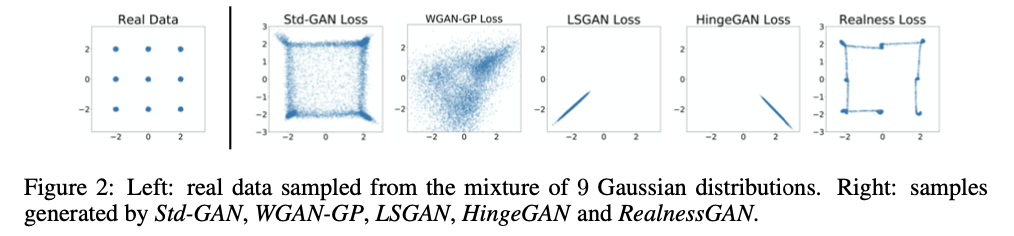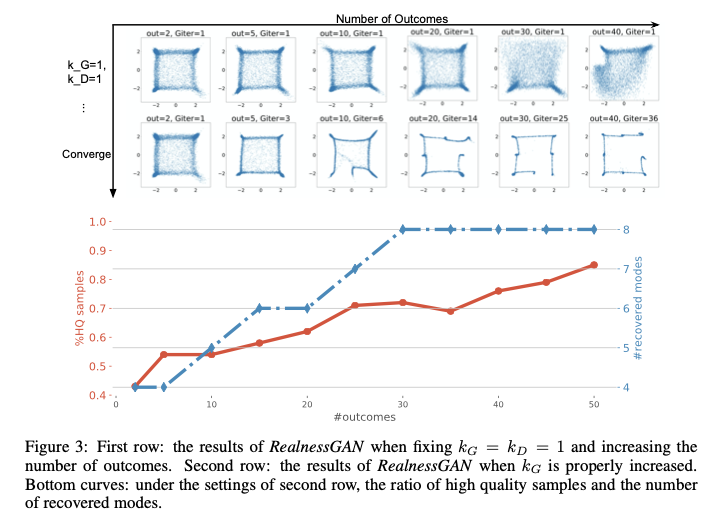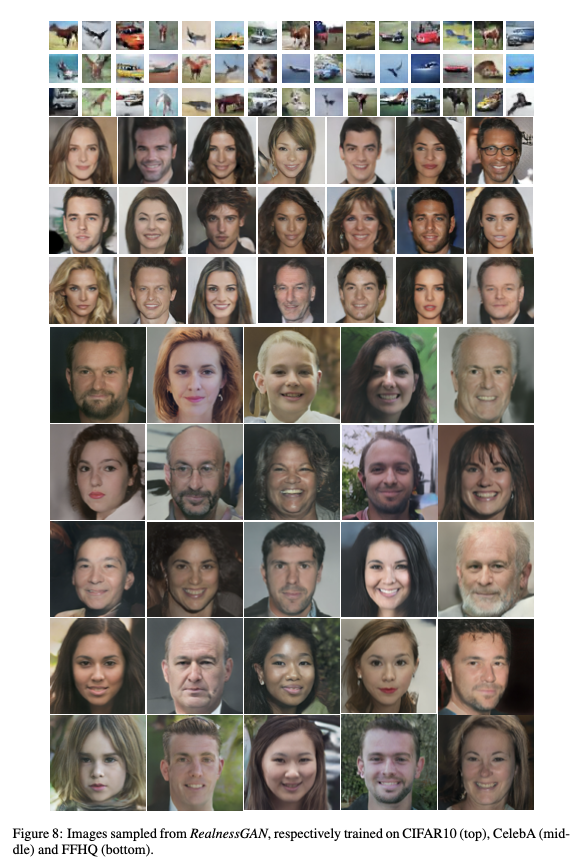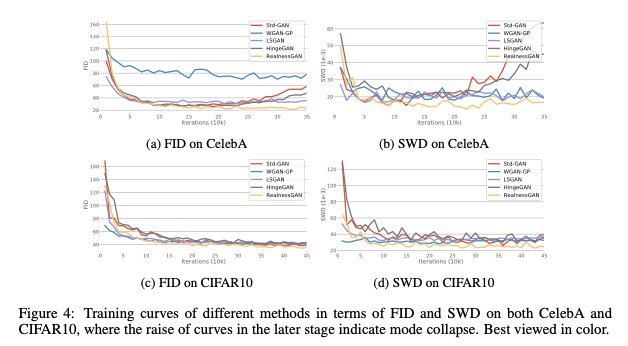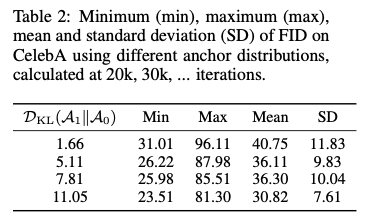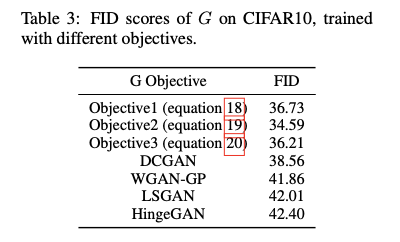# 总结

RealnessGAN从分布的角度扩展了生成对抗网络的逼真性，以判别输出分布替代了标准GAN中的单标量判别输出，此时判别器会从多个角度估算真实性，从而为生成器提供更多信息指导。同时这种判别输出分布的方式可以推广到更多的一般性GAN的训练上，从理论和实验分析上可以得到该方法的有效性，对于GAN的发展起到了推助作用。

# 参考文献

 Ledig C, Theis L, Huszár F, et al. Photo-realistic single image super-resolution using a generative adversarial network[C]//Proceedings of the IEEE conference on computer vision and pattern recognition. 2017: 4681-4690.

 Zhang Z, Yu J. STDGAN: ResBlock Based Generative Adversarial Nets Using Spectral Normalization and Two Different Discriminators[C]//Proceedings of the 27th ACM International Conference on Multimedia. 2019: 674-682.

 Gulrajani I, Ahmed F, Arjovsky M, et al. Improved training of wasserstein gans[C]//Advances in neural information processing systems. 2017: 5767-5777.

 Mao X, Li Q, Xie H, et al. Least squares generative adversarial networks[C]//Proceedings of the IEEE International Conference on Computer Vision. 2017: 2794-2802.

 Junbo Zhao, Michael Mathieu, and Yann LeCun. Energy-based generative adversarial network. In ICLR, 2017.